Definitions of Square Dance Calls and Concepts

Index -->  Plus  |  A1  |  A2  |  C1  |  C2  |  C3A  |  C3B  |  C4  |  NOL  |
Definitions (Text Only) -->  Plus  |  A1  |  A2  |  C1  |  C2  |  C3A  |  C3B  |  C4  |  NOL  |
 Find call:

\$BMM!9\$J(B formation \$B\$+\$i(B.

Any Tagging call (\$B\$^\$?\$O(B Tagging call \$B\$N1~MQ(B) \$B\$r(B, 1/2 Tag \$B\$N0LCV\$^\$G9T\$\$(B; Tandem Arm Turn 1/4 (\$B\$9\$J\$o\$A(B, Split Counter Rotate 1/4) \$B\$r\$7(B; any Tagging call \$B\$r=*\$o\$i\$;\$^\$9(B.

Reflected Flip The Line: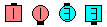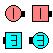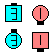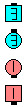Reflected Flip The Line\$B\$NA0(B 1/2 Flip The Line\$B\$N8e(B Tandem Arm Turn 1/4(Box Counter Rotate 1/4)\$B\$N8e(B Complete The Flip The Line \$B\$N8e(B (\$B=*\$o\$j(B)
Reflected Track 3: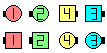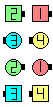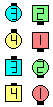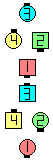Reflected Track 3\$B\$NA0(B Track 2\$B\$N8e(B Tandem Arm Turn 1/4(Split Counter Rotate 1/4)\$B\$N8e(B Extend\$B\$N8e(B (\$B=*\$o\$j(B)

\$BCm
• Reflected (\$BH?Tagging call \$B\$r;O\$a\$F??\$CD>\$0?J\$s\$G\$\$\$k\$H\$-\$K(B, \$B2>A[\$NJI\$K\$V\$D\$+\$j(B, 90° \$BH?
• Track 3 \$B\$^\$?\$O(B Invert The Column \$B\$N\$h\$&\$J%3!<%k\$G\$O(B, \$B%3!<%k\$r9T\$&\$K\$O(B 8 \$B?M\$N%@%s%5!<\$,I,MW\$G\$9\$,(B, Tagging \$B\$NItJ,\$G\$O(B, 4 \$B?M\$@\$1(B (4 \$B?M\$,(B 2 \$BAH(B) \$B\$G9T\$\$\$^\$9(B.
• Reflected \$B\$N0lHLE*\$JDj5A\$O(B, \$BM?\$(\$i\$l\$?%3!<%k\$r(B 1/2 Tag \$B\$N0LCV\$^\$G9T\$\$(B; \$B%3!<%k\$N(B Tagging \$B\$NItJ,\$r9T\$&%@%s%5!<\$N?t\$N>l=j\$G(B Counter Rotate 1/4 \$B\$r9T\$\$(B; \$BM?\$(\$i\$l\$?%3!<%k\$r=*\$o\$i\$;\$^\$9(B.

Reflected Partner Tag:
\$B%+%C%W%k\$^\$?\$O(B Mini-Wave \$B\$+\$i(B. 1/4 In and Touch (\$B\$3\$l\$,(B Partner 1/2 Tag) \$B\$r\$7(B; Arm Turn 1/4 (Tagging \$B\$NItJ,\$G\$O(B 2 \$B?M\$7\$+\$\$\$J\$\$\$N\$G(B) \$B\$r\$7(B; Step Thru (Back-to-Back \$B\$H\$J\$j\$^\$9(B) \$B\$r\$7\$^\$9(B.
Line of 6 Reflected Tag The Line:
6 \$B?M\$N(B Line \$B\$+\$i(B. Line of 6 1/2 Tag \$B\$r\$7(B; Tandems of 3 Arm Turn 1/4 \$B\$r\$7(B; \$BA40w\$G(B Tag \$B\$r=*\$o\$i\$;(B Tandems of 3 \$B\$,(B Back-to-Back \$B\$H\$J\$j\$^\$9(B.
• Reflected Partner Tag: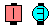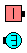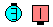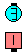Reflected Partner Tag\$B\$NA0(B Partner 1/2 Tag\$B\$N8e(B Arm Turn 1/4\$B\$N8e(B Step Thru\$B\$N8e(B (\$B=*\$o\$j(B)
Center Line Of 6 Reflected 1/2 Tag: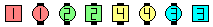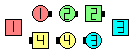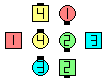Center Line of 6 Reflected 1/2 Tag\$B\$NA0(B Center Line of 6 1/2 Tag\$B\$N8e(B Center 6 Counter Rotate 1/4\$B\$N8e(B (\$B=*\$o\$j(B)

\$BTagging Calls.Choreography for Reflected ConceptComments? Questions? Suggestions?

https://www.ceder.net/def/reflected.php?language=japan&level=C3B
08-June-2023 01:47:19